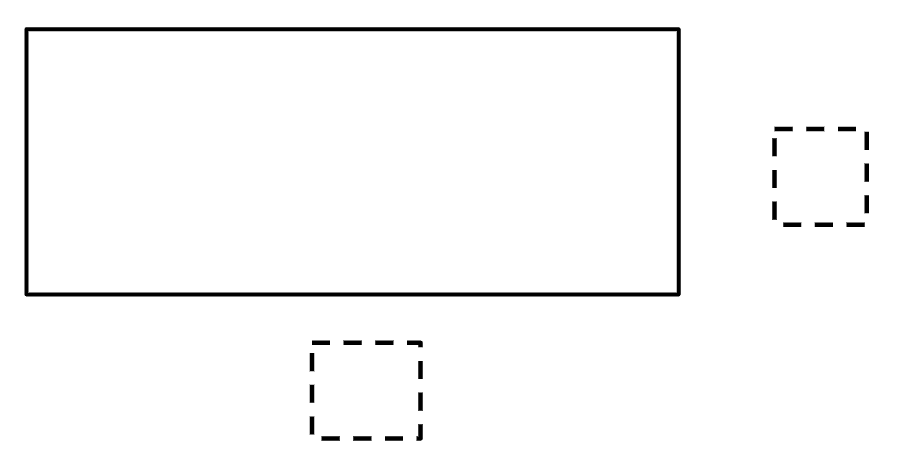# Area of a Rectangle

Directions: Using the digits 1 – 9, at most once each, fill in the blanks to make it so that the value for the area of the rectangle (in square units) is greater that the value for the perimeter (in linear units). What is the greatest difference you can find between the area and perimeter? What is the least difference you can find?### Hint

The way to find the area of a quadrilateral is b(base) * h(height).

One answer is a base of 6 and a height of 5, and an area of 30, and a perimeter of 22.

Source: Owen Kaplinsky

## Product Close to 1,000

Directions: Using the digits 1 to 9 at most one time each, place a digit …

1.2.